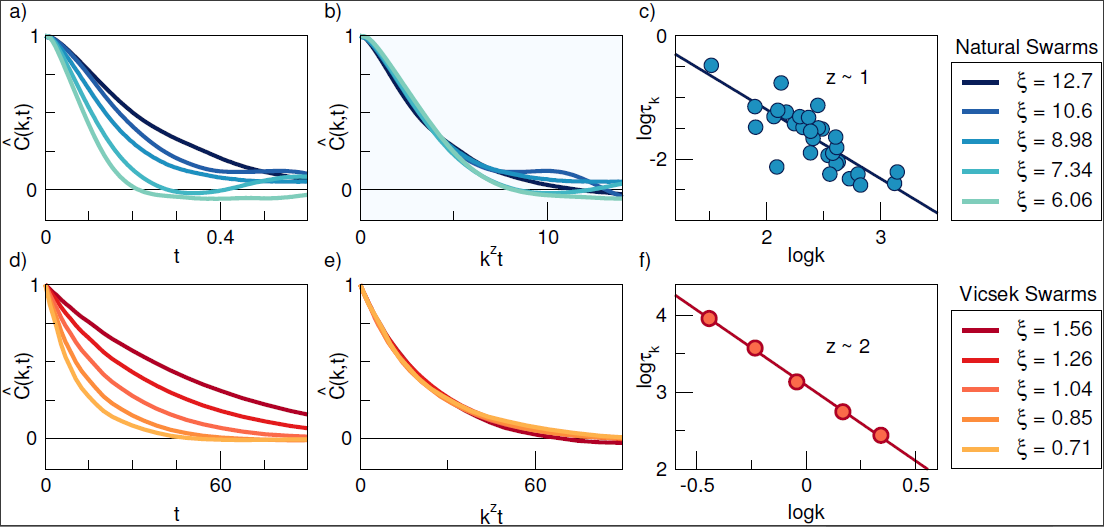# How do birds flock and insects swarm: the Problem with current models

if you haven't seen the first part of this introduction, absolutely go check it out for context.

In this post we'll unravel how does the delay in a group of animals affect the group, and what should we understand under said delay (keyword: slowpoke). To get there, let's analyse the last two paragraphs of the introduction to my thesis, where we'll see what problems can be spotted in previous research, and motivate ourselves to look for solutions.

Both classic and dynamic scaling have previously been shown to work both for natural swarms, as well as for the Vicsek model. But when Cavagna et al. tried to compare the behaviour of natural swarms to that of the Vicsek model, two unexpected problems arose. First, in the relaxation of the spatio-temporal correlation function, non-dissipative modes were found in natural swarms, resulting in an underdamped correlation function. This suggests a sort of behavioural inertia in swarms, not present in the classic Vicsek model. The other difference comes in the value of the dynamic critical exponent $z$ resulting from testing the dynamic scaling hypothesis. For natural swarms $z$ assumes the value of approximately $1$, while for the Vicsek model $z\approx2$.

Firstly, there is research out there that concentrates on collecting data from real groups of animals that behave in a certain way. This data is then analysed with our friendly neighbourhood statistical tools, such as different correlation functions. Through that analysis a control parameter for that type of group is found - namely the critical exponent, which allows us to use the scaling hypothesis to predict the behaviour of groups of different sizes, or other variables that we know the system depends on.

Secondly, there is research that concentrates on trying to simulate the behaviour of these groups of animals using different models - like the Vicsek model, which we discussed previously. By simulating that data, we can then analyse it the same way as we would a real group of animals, and see if the results are similar, and if they differ - how exactly. This can tell us how to improve the model (and by extension our theories), and iterate again.from Dynamic scaling in natural swarms - Cavagna et al. (2017)

Previous research of simulated data of the Vicsek model was promising, in the sense that for being such a simple model (we will discuss what the model actually entails in the next post) it still replicated many of the analysis results for different animal groups. But in recent research it started to deviate slightly from experimental data in two major ways. One way that it differs is in the shape of the dynamic correlation function. In experiment it suggests that when our old friend Alice the midge changes it's direction, Bob's trajectory changes as if it has inertia - it doesn't turn immediately to it's new trajectory according to Alice's, but instead takes a bit of time to readjust to its new direction. Meanwhile in the Vicsek model there is no such thing as inertia. Once Alice turns, Bob immediately acts and turns as well.

Another problem which arises (and could be connected to the previous one) is the calculated critical exponent - our control parameter for the scaling hypothesis. It takes on different values for the dynamic correlation function of experimental data versus our simulated Vicsek model data. By solving our first problem though, we can check whether our second problem gets better, worse, or maybe resolves itself.

Delay seems like an inevitable part of these biological systems. Even though some preliminary work has been done on the 2D Vicsek model with time delay, the dynamical scaling hypothesis has not yet been tested on it, especially in three dimensions. The shape of the spatio-temporal correlation function in natural swarms suggests that adding a sensorial time delay to the change in direction of an individual particle in the Vicsek model could potentially simulate the behavioural inertia, and lead to results closer to those found in experiments. In this work, this hypothesis will be tested by modifying the update equations of the Vicsek model, and simulating it in the near-critical regime to get a new value for $z$ and observe any possible change in the shape of the correlation function.

So the question arises - how do we solve our lack-of-inertia problem in the Vicsek model? It has no inertia in its equations of motion after all. One possible thing to test is adding a time delay to the reaction of animals in the group to changes in its neighbours. What this means, is that when Alice turns, Bob continues to fly in its old trajectory for a set amount of time (time delay), and only when that time passes, does he turn to his newly decided direction. This can potentially simulate inertial behaviour, and we can test whether this new model still obeys the hypotheses of scale invariability. So let's make a plan of action:

1. Change the Vicsek model's equations of motion so that they have time delay included in them
2. Simulate this new model for different system sizes and different values of time delay
3. Analyse that data, to see if it obeys the scaling hypothesis, and if the results are any closer to experiment than the ones of the standard Vicsek model

So, this is what we will discuss in the upcoming posts. We will see what the model is like, and discuss how one goes around to write a simulator for this model. This will be a mostly coding related post, where we concentrate on the technical aspects of computer simulations and statistical analysis. Lastly, we will discuss our results - the physics of it all. This will be our conclusion on the matter, where we see what our model revealed about the world of collective behaviour of natural systems.